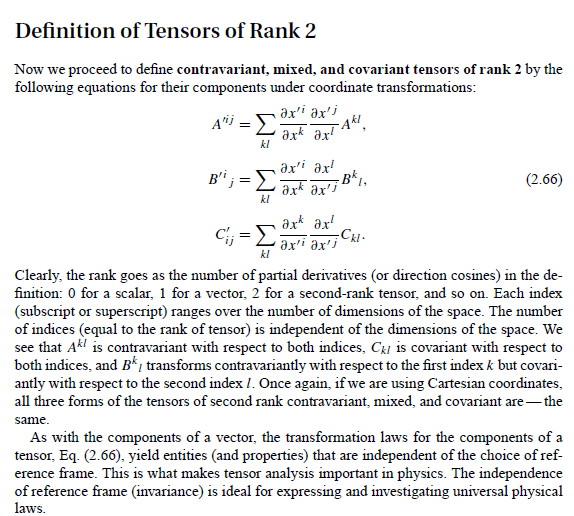# Math Methods for Physicists by Arfken Questions?

Hello Big Minds,

I am reading through Math Methods for Physicists by Arfken and Weber 6th ed --Tensor Analysis.

-----------------------------------------------------------------------------------------------------------------------------------------------------------
Note1:
In page 133, in the foot note it is said that "In N-dimensional space a tensor of rank n has N^n componenets"...in page 135 I understood from the written text that Contravariant, Covariant and Mixed tensors set of equations (2.66) works independent of reference frame and space dimension.
Q1: how can one use equations 2.66 to transform from 4-D (Minkowiski space-time) )to 11-D dimension (as one proposal of String theory) for instance while both sides of Tensors in equations 2.66 must have the same rank (this case of rank 2)? Simply speaking, the number of Tensor's indices depend on the rank of the tensor and the rank of the tensor depends on the dimension but equations 2.66 are independent of the dimension...how is that?
plus his indices of coordinate transformation of contravariant were defined in the subscript first 2.62a but they apear in the superscript in the first equation of 2.66...why? (they must be the same as in this link)

Page(s):-----------------------------------------------------------------------------------------------------------------------------------------------------------

Best regards,

Abolaban

Last edited:

jedishrfu
Mentor
I think you're confusing rank with dimension.

A tensor of rank 2 is like a matrix. In 3 dimensions the matrix would be 3x3, for 4 dimensions 4x4 and for 11 dimensions it would be 11x11.

The dimension comes into play in a tensor in the value of the indices ie I and j values for 3 dimensions would be { 1, 2, 3 } and for 11 would be { 1, 2, 3, ... 11 }